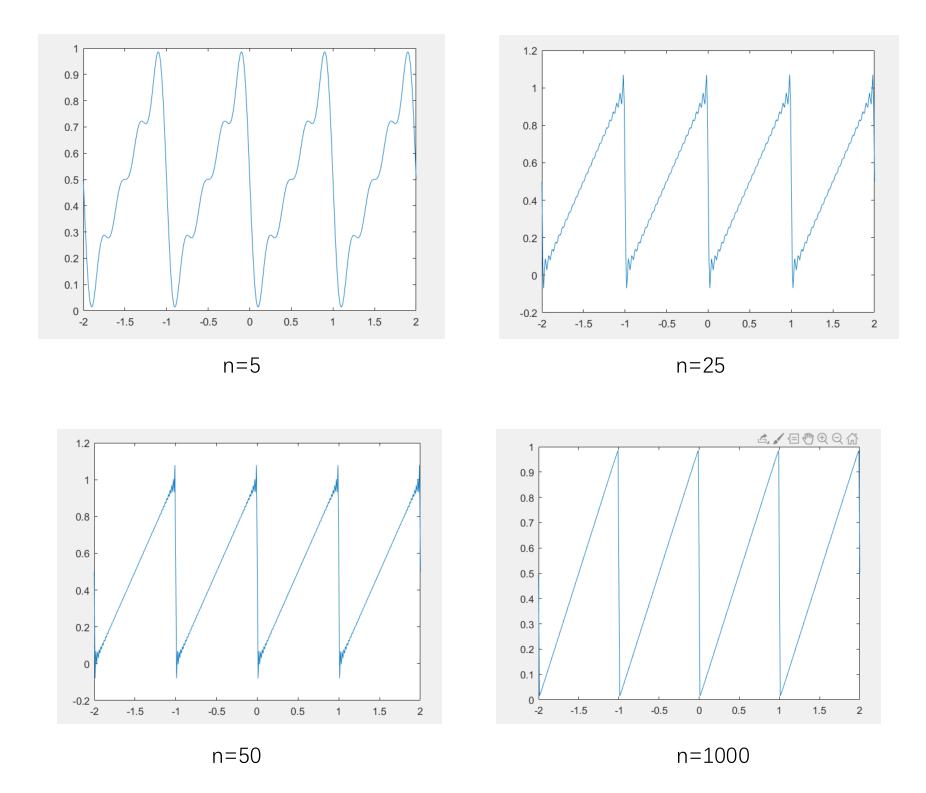# 引入

f(t) = t - [t] , [t]为不大于t的最大整数

# 原理

## 1. 复数表示

f(t) = t - [t] , [t]为不大于t的最大整数

a k = 1 T ∫ 0 T f ( t ) e − j k ω 0 t d ⁡ t a_k=\frac1T\int_0^Tf\left(t\right)e^{-jk\omega_0t}\operatorname dt

f ( t ) ^ = ∑ k = − N N a k e j k w 0 t \widehat{f(t)}={\textstyle\sum_{k=-N}^N}a_ke^{jkw_0t}

## 2. 三角函数

f ( t ) = a 0 2 + ∑ n = 1 ∞ a n cos ⁡ ( n π L t ) + ∑ n = 1 + ∞ b n sin ⁡ ( n π L t ) f(t)=\frac{a_0}2+{\textstyle\sum_{n=1}^\infty}a_n\cos(\frac{n\pi}Lt)+{\textstyle\sum_{n=1}^{+\infty}}b_n\sin(\frac{n\pi}Lt)

a n = 1 L ∫ − L L f ( t ) cos ⁡ n π L t d ⁡ t a_n=\frac1L\int_{-L}^Lf(t)\cos\frac{n\pi}Lt\operatorname dt
b n = 1 L ∫ − L L f ( t ) sin ⁡ n π L t d ⁡ t b_n=\frac1L\int_{-L}^Lf(t)\sin\frac{n\pi}Lt\operatorname dt

a n = { 2 ∫ 0 1 t cos ⁡ ( 2 n π t ) d t = 0 n ≠ 0 1 n = 0 a_n=\left\{\begin{array}{lc}2\int_0^1t\cos(2n\pi t)dt=0&n\neq0\\1&n=0\end{array}\right.
b n = 2 ∫ 0 1 t sin ⁡ ( 2 n π t ) d t = − 1 n π bn=2\int_0^1t\sin(2n\pi t)dt=-\frac1{n\pi}

f ( t ) ^ = 1 + ∑ n = 1 ∞ − 1 n π sin ⁡ ( 2 n π t ) \widehat{f(t)}=1+{\textstyle\sum_{n=1}^\infty}{\textstyle\frac{-1}{n\pi}}\sin(2n\pi t)

# matlab代码

## 三角函数

### 方法1（不推荐，运行时间很长）

clear;
x = -3:0.01:3;
N = input('please enter the N')
y = g(N);  %N为相加的项数
plot(x,y);

%定义累加函数，其变量N决定相加项数的多少
function y=g(N)
x = -3:0.01:3;
y = zeros(1,length(x))
y = a(0)/2+y;
for k = 1:N
ai= a(k).*cos(2*k*pi.*x)+b(k).*sin(2*k*pi.*x);
y = y + ai;  %各项累加
end
end

%定义求an的函数
function ak = a(k)
syms t;
ak = 2*int(t*cos(2*k*pi*t),t,0,1);
end

%定义求bn的函数
function bk = b(k)
syms t;
bk = 2*int(t*sin(2*k*pi*t),t,0,1);
end


### 方法2

clear
n = input("please enter the n"); %取无穷级数的前K项叠加来近似f(t)
t = -2:0.01:2;
for k = 2:n
f(k,:)=(-1)/pi/(k-1)*sin(2*pi*(k-1)*t);
end
f(1,:) = 1/2;  %加入a0的值
F = sum(f,1);
plot(t,F);


# 运行结果11-06
03-291954
12-303860
04-143117
05-09
05-172万+
11-149268
11-141万+
03-023万+
03-192980
11-20484
03-26616
03-301458
09-271206
07-092万+
03-173130
03-243616
04-142616
05-279504

### “相关推荐”对你有帮助么？

•非常没帮助
•没帮助
•一般
•有帮助
•非常有帮助被折叠的  条评论 为什么被折叠?到【灌水乐园】发言Hu4459

¥2 ¥4 ¥6 ¥10 ¥20余额支付 (余额：-- )扫码支付获取中扫码支付点击重新获取扫码支付1.余额是钱包充值的虚拟货币，按照1:1的比例进行支付金额的抵扣。
2.余额无法直接购买下载，可以购买VIP、C币套餐、付费专栏及课程。余额充值